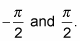##### Trigonometry Workbook For DummiesA function that has an inverse has exactly one output (belonging to the range) for every input (belonging to the domain), and vice versa. To keep inverse trig functions consistent with this definition, you have to designate ranges for them that will take care of all the possible input values and not have any duplication.

The output values of the inverse trig functions are all angles — in either degrees or radians — and they’re the answer to the question, “Which angle gives me this number?” In general, the output angles for the individual inverse functions are paired up as angles in Quadrants I and II or angles in Quadrants I and IV. The quadrants are selected this way for the inverse trig functions because the pairs are adjacent quadrants, allowing for both positive and negative entries. The notation for these inverse functions uses capital letters.

## Domain and range of inverse sine function

The domain for Sin1 x, or Arcsin x, is from –1 to 1. In mathematical notation, the domain or input values, the x’s, fit into the expression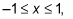because no matter what angle measure you put into the sine function, the output is restricted to these values. The range, or output, for Sin1 x is all angles from –90 to 90 degrees or, in radians,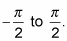If the output is the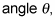then you write these expressions as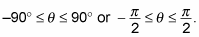The outputs are angles in the adjacent Quadrants I and IV, because the sine is positive in the first quadrant and negative in the second quadrant. Those angles cover all the possible input values.

## Domain and range of inverse cosine function

The domain for Cos1 x, or Arccos x, is from –1 to 1, just like the inverse sine function. So the x (or input) values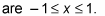The range for Cos1 x consists of all angles from 0 to 180 degrees or, in radians,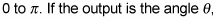then you write these expressions as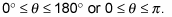The outputs are angles in the adjacent Quadrants I and II, because the cosine is positive in the first quadrant and negative in the second quadrant. Those angles cover all the possible input values for the function.

## Domain and range of inverse tangent function

The domain for Tan1 x, or Arctan x, is all real numbers — numbers from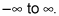This is because the output of the tangent function, this function’s inverse, includes all numbers, without any bounds. The range, or output, of Tan1 x is angles between –90 and 90 degrees or, in radians, between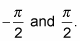One important note is that the range doesn’t include those beginning and ending angles; the tangent function isn’t defined for –90 or 90 degrees. The range of Tan1 x includes all the angles in the adjacent Quadrants I and IV, except for the two angles with terminal sides on the y-axis.

## Domain and range of inverse cotangent function

The domain of Cot1 x, or Arccot x, is the same as that of the inverse tangent function. The domain includes all real numbers. The range, though, is different — it includes all angles between 0 and 180 degrees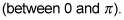So any angle in Quadrants I and II is included in the range, except for those with terminal sides on the x-axis. Those two angles aren’t in the domain of the cotangent function, so they aren’t in the range of the inverse.

## Domain and range of inverse secant function

The domain of Sec1 x, or Arcsec x, consists of all the numbers from 1 on up plus all the numbers from –1 on down. Letting x be the input, you write this expression as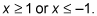In other words, the domain includes all the numbers fromexcept for the numbers between –1 and 1. The range of Sec1 x is all the angles between 0 and 180 degrees except for 90 degrees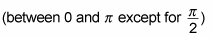— meaning all angles in Quadrants I and II, with the exception of 90 degrees, or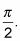## Domain and range of inverse cosecant function

The domain of Csc1 x, or Arccsc x, is the same as that for the inverse secant function, all the numbers from 1 on up plus all the numbers from –1 on down. The range is different, though — it includes all angles between –90 and 90 degrees except for 0 degrees or, in radians, between